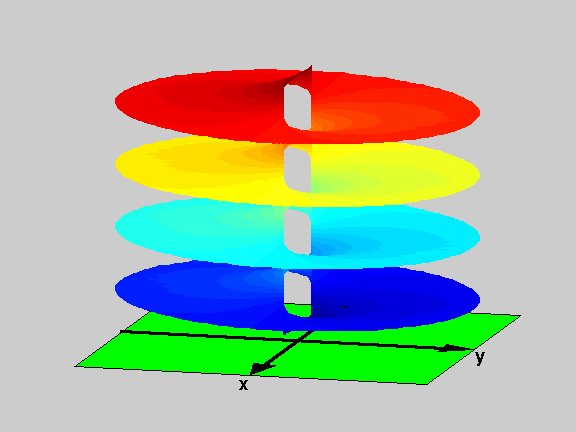18.04 | Fall 1999 | Undergraduate

# Complex Variables with Applications

Study Materials

## Riemann Surfaces: f(z)=log((z+1)/(z-1)) View 1

(All images created with MATLAB® software)

Riemann Surface for f(z) = log((z+1)/(z-1)). The picture shows only four levels of the surface, which extends up and down with infinitely many similar levels (notice the similarity with a parking building, with connecting ramps between levels at the center). The only singularities in the surface are at the branch points: z=1 and z=-1 (the edges of of the connecting ramps in the parking building analogy).

Picture obtained using the script RiemannSur in the Athena 18.04 MATLAB® Toolkit.Figure 1: Riemann Surface of the function f(z)=log((z+1)/(z-1))